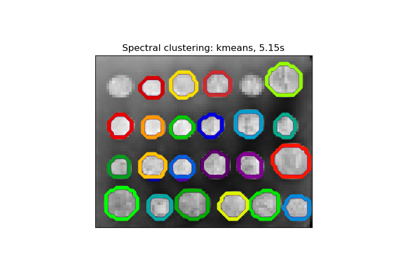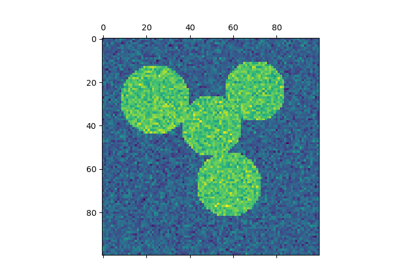# sklearn.cluster.spectral_clustering¶

sklearn.cluster.spectral_clustering(affinity, n_clusters=8, n_components=None, eigen_solver=None, random_state=None, n_init=10, eigen_tol=0.0, assign_labels=’kmeans’)[source]

Apply clustering to a projection of the normalized Laplacian.

In practice Spectral Clustering is very useful when the structure of the individual clusters is highly non-convex or more generally when a measure of the center and spread of the cluster is not a suitable description of the complete cluster. For instance, when clusters are nested circles on the 2D plane.

If affinity is the adjacency matrix of a graph, this method can be used to find normalized graph cuts.

Read more in the User Guide.

Parameters: affinity : array-like or sparse matrix, shape: (n_samples, n_samples) The affinity matrix describing the relationship of the samples to embed. Must be symmetric. Possible examples: adjacency matrix of a graph, heat kernel of the pairwise distance matrix of the samples, symmetric k-nearest neighbours connectivity matrix of the samples. n_clusters : integer, optional Number of clusters to extract. n_components : integer, optional, default is n_clusters Number of eigen vectors to use for the spectral embedding eigen_solver : {None, ‘arpack’, ‘lobpcg’, or ‘amg’} The eigenvalue decomposition strategy to use. AMG requires pyamg to be installed. It can be faster on very large, sparse problems, but may also lead to instabilities random_state : int, RandomState instance or None (default) A pseudo random number generator used for the initialization of the lobpcg eigen vectors decomposition when eigen_solver == ‘amg’ and by the K-Means initialization. Use an int to make the randomness deterministic. See Glossary. n_init : int, optional, default: 10 Number of time the k-means algorithm will be run with different centroid seeds. The final results will be the best output of n_init consecutive runs in terms of inertia. eigen_tol : float, optional, default: 0.0 Stopping criterion for eigendecomposition of the Laplacian matrix when using arpack eigen_solver. assign_labels : {‘kmeans’, ‘discretize’}, default: ‘kmeans’ The strategy to use to assign labels in the embedding space. There are two ways to assign labels after the laplacian embedding. k-means can be applied and is a popular choice. But it can also be sensitive to initialization. Discretization is another approach which is less sensitive to random initialization. See the ‘Multiclass spectral clustering’ paper referenced below for more details on the discretization approach. labels : array of integers, shape: n_samples The labels of the clusters.

Notes

The graph should contain only one connect component, elsewhere the results make little sense.

This algorithm solves the normalized cut for k=2: it is a normalized spectral clustering.

References

## Examples using sklearn.cluster.spectral_clustering¶Segmenting the picture of greek coins in regionsSpectral clustering for image segmentation